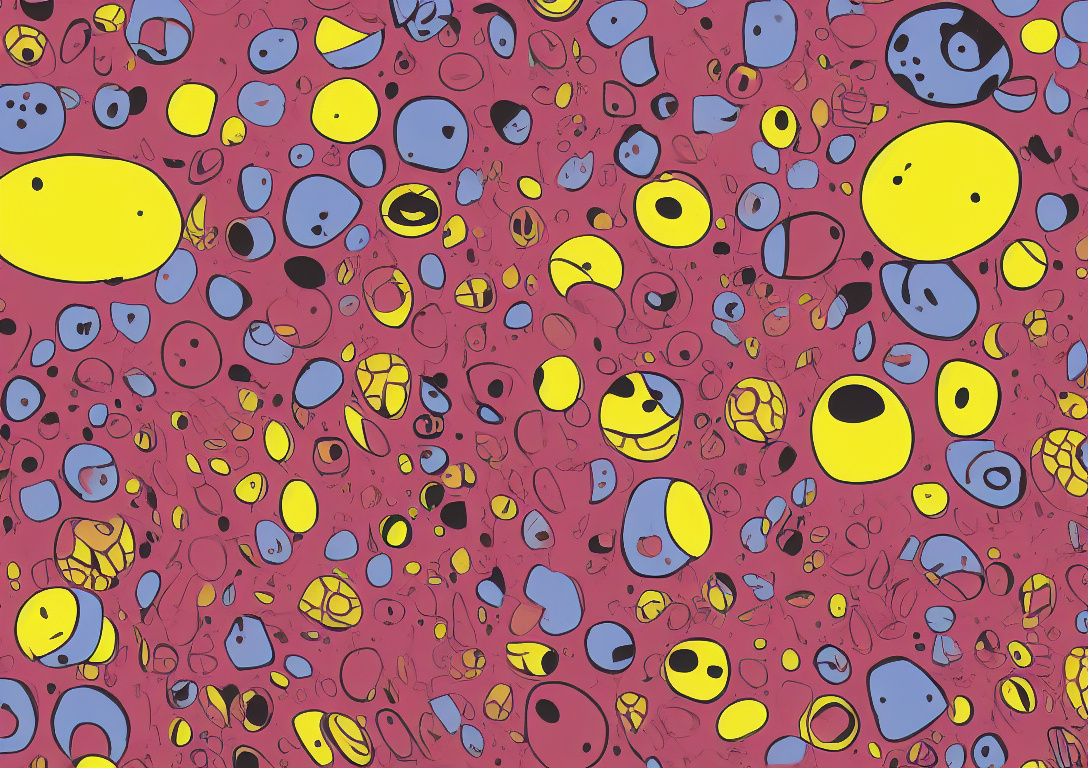# Finding the location of objects in a selection for fabric.jsSelections are just groups. In fabric.js < 6, the objects are moved from the canvas into the selection group. This causes typical object position to be relative to the selection instead of the position on the canvas.

To find the location of objects, we can use the `obj.calcTransformMatrix()`. This is the true position of the object on the canvas.

``````const canvas = new fabric.Canvas(document.createElement("canvas"));

const rect = new fabric.Rect({
width: 10,
height: 10,
left: 100,
top: 100,
originX: 0.5,
originY: 0.5,
strokeWidth: 0,
});
const rect2 = new fabric.Rect({
width: 10,
height: 10,
left: 200,
top: 200,
originX: 0.5,
originY: 0.5,
strokeWidth: 0,
});

const selection = new fabric.ActiveSelection([rect, rect2], { canvas });

console.log(selection.calcTransformMatrix());
// [ 1, 0, 0, 1, 150, 150 ]

console.log(selection.calcOwnMatrix());
// [ 1, 0, 0, 1, 150, 150 ]

selection.getObjects().forEach((obj) => {
console.log(obj.calcTransformMatrix());
// [ 1, 0, 0, 1, 100, 100 ]
// [ 1, 0, 0, 1, 200, 200 ]
console.log(obj.calcOwnMatrix());
// [ 1, 0, 0, 1, -50, -50 ]
// [ 1, 0, 0, 1, 50, 50 ]
});
``````

In this example we can see the relative locations on `calcOwnMatrix` vs `calcTransformMatrix`. Notice how the calcTransformMatrix stays right on the origin location based on `originX ` and `originY` and `left` and `top`. Selection will set their transform matrix to be the center as well.

If we do a slight rotation of our selection, we can see how that reflects.

``````selection.angle = 10;
``````

And then see how our matrices form up.

``````console.log(selection.calcTransformMatrix());
// [
//   0.984807753012208,
//   0.17364817766693033,
//   -0.17364817766693033,
//   0.984807753012208,
//   139.61377664399026,
//   158.71507618735262
// ]

console.log(selection.calcOwnMatrix());
// [
//   0.984807753012208,
//   0.17364817766693033,
//   -0.17364817766693033,
//   0.984807753012208,
//   139.61377664399026,
//   158.71507618735262
// ]

selection.getObjects().forEach((obj) => {
console.log(obj.calcTransformMatrix());
// [
//   0.984807753012208,
//   0.17364817766693033,
//   -0.17364817766693033,
//   0.984807753012208,
//   99.05579787672639,
//   100.7922796533957
// ]
// [
//   0.984807753012208,
//   0.17364817766693033,
//   -0.17364817766693033,
//   0.984807753012208,
//   180.17175541125414,
//   216.63787272130955
// ]
console.log(obj.calcOwnMatrix());
// [ 1, 0, 0, 1, -50, -50 ]
// [ 1, 0, 0, 1, 50, 50 ]
});
``````

Here you can see the selection transform matrix changes, and also applies the new location to the transform matrices of the children objects, but the relative location remains the same.

fabric.js v6 has a new function, `applyTransformsToObject` that can be applied to an object to have it apply these transforms on it. Or use these values to compare locations to another.

Since the objects are inside a selection, you wouldn’t want to apply the true position of the object but need to multiply this matrix of the invert of the selection position to get the relative position.

``````console.log(
fabric.util.multiplyTransformMatrices(
fabric.util.invertTransform(selection.calcTransformMatrix()),
obj.calcTransformMatrix()
)
);
// [
//   1.0000000000000002,
//   2.7755575615628914e-17,
//   -2.7755575615628914e-17,
//   1.0000000000000002,
//   50,
//   50.00000000000003
// ]
``````

To go from selection transform matrix to the relative location we can multiply the inverted transform of the selection matrix onto the objects transform matrix. Basically remove the selection transform the make it relative positionally. Note the values are slightly off due to floating points. Compare to the `calcOwnMatrix()` values for the objects.

## Conclusion

• `calcTransformMatrix()` is the true transforms in relation to the canvas

• `calcOwnMatrix()` is the transforms in relation to the parent (canvas or group)

• new `applyTransformsToObject` to apply a new transform matrix on an object